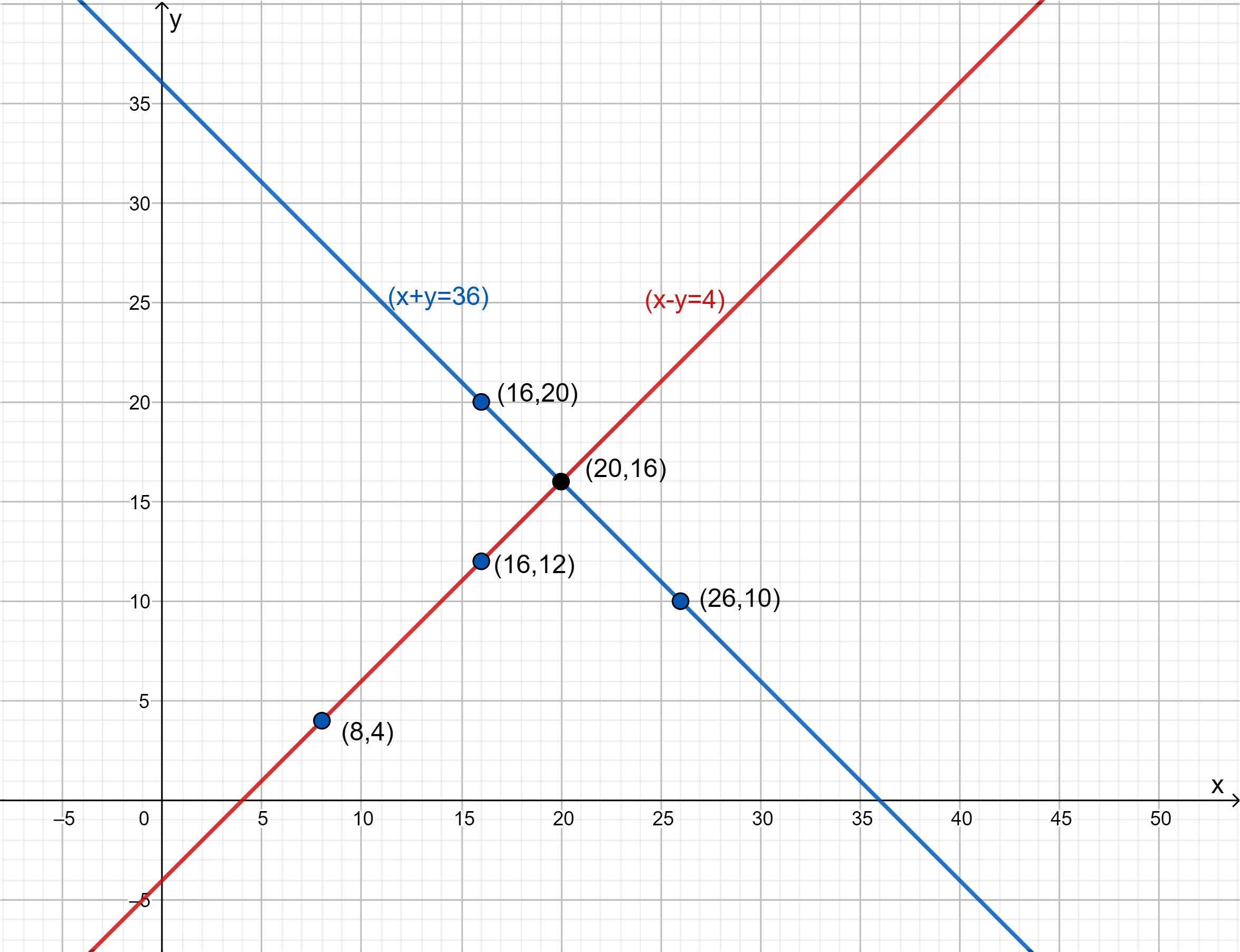In the verge of coronavirus pandemic, we are providing FREE access to our entire Online Curriculum to ensure Learning Doesn't STOP!

# Ex.3.2 Q5 Pair of Linear Equations in Two Variables Solution - NCERT Maths Class 10

Go back to  'Ex.3.2'

## Question

Half the perimeter of a rectangular garden, whose length is $$4 \,\rm{m}$$ more than its width, is $$36\,\rm{ m.}$$ Find the dimensions of the garden.

Video Solution
Pair Of Linear Equations In Two Variables
Ex 3.2 | Question 5

## Text Solution

What is Known?

(i) Half the perimeter of rectangular garden $$= 36{\rm{\,m }}$$

(ii) Length is $$4{\rm{ \,m}}$$ more than width

What is Unknown?

Dimensions of the garden

Reasoning:

Assuming length of the garden as $$x$$ and width of the garden as $$y,$$ two linear equations can be formed for the known data.

Perimeter of rectangle $$=$$ 2(Length $$+$$ Breadth)

Steps:

Let the length of the garden be $$x$$ and breadth be $$y$$

Then,

\begin{align}x& = y + 4\\ x - y &= 4\\y &= x - 4\end{align}

 $$x$$ $$8$$ $$16$$ $$y = x - 4$$ $$4$$ $$12$$

Half perimeter of the rectangle be $$x + y = 36$$

$$y = 36 - x$$

 $$x$$ $$16$$ $$26$$ $$y = x - 4$$ $$20$$ $$10$$Length $$x = 20{\rm{\; m}}$$
Breadth $$y = 16{\rm{\; m}}$$